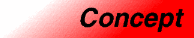# StrictWeakOrderingCategory: functors Component type: concept

## Description

A Strict Weak Ordering is a BinaryPredicate that compares two objects, returning `true` if the first precedes the second. This predicate must satisfy the standard mathematical definition of a strict weak ordering. The precise requirements are stated below, but what they roughly mean is that a Strict Weak Ordering has to behave the way that "less than" behaves: if `a` is less than `b` then `b` is not less than `a`, if `a` is less than `b` and `b` is less than `c` then `a` is less than `c`, and so on.

## Associated types

 First argument type The type of the Strict Weak Ordering's first argument. Second argument type The type of the Strict Weak Ordering's second argument. The first argument type and second argument type must be the same. Result type The type returned when the Strict Weak Ordering is called. The result type must be convertible to `bool`.

## Notation

 `F` A type that is a model of Strict Weak Ordering `X` The type of Strict Weak Ordering's arguments. `f` Object of type `F` `x`, `y`, `z` Object of type `X`

## Definitions

• Two objects `x` and `y` are equivalent if both `f(x, y)` and `f(y, x)` are false. Note that an object is always (by the irreflexivity invariant) equivalent to itself.

## Valid expressions

None, except for those defined in the BinaryPredicate requirements.

## Expression semantics

Name Expression Precondition Semantics Postcondition
Function call `f(x, y)` The ordered pair `(x,y)` is in the domain of `f` Returns `true` if `x` precedes `y`, and `false` otherwise The result is either `true` or `false`

## Invariants

 Irreflexivity `f(x, x)` must be `false`. Antisymmetry `f(x, y)` implies `!f(y, x)` Transitivity `f(x, y)` and `f(y, z)` imply `f(x, z)`. Transitivity of equivalence Equivalence (as defined above) is transitive: if `x` is equivalent to `y` and `y` is equivalent to `z`, then `x` is equivalent to `z`. (This implies that equivalence does in fact satisfy the mathematical definition of an equivalence relation.) 

## Models

• `less<int>`
• `less<double>`
• `greater<int>`
• `greater<double>`